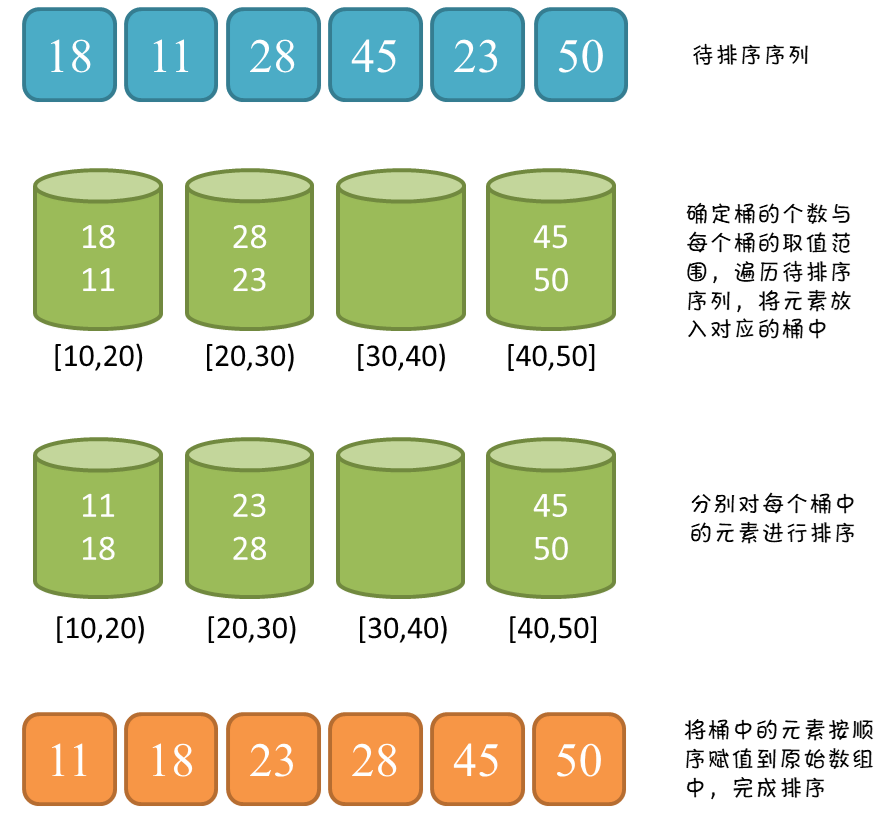# 桶排序（Bucket sort）

## 算法流程

1. 设置一个定量的数组当作空桶子。

2. 寻访序列，并且把项目一个一个放到对应的桶子去。

3. 对每个不是空的桶子进行排序。

4. 从不是空的桶子里把项目再放回原来的序列中。# java 实现

## 实现

package com.github.houbb.sort.core.api;

import com.github.houbb.log.integration.core.Log;
import com.github.houbb.log.integration.core.LogFactory;

import java.util.ArrayList;
import java.util.Collections;
import java.util.List;

/**
* 桶排序
*
* @author binbin.hou
* @since 0.0.9
*/
public class BucketSort extends AbstractSort {

private static final Log log = LogFactory.getLog(BucketSort.class);

@Override
@SuppressWarnings("all")
public void doSort(List original) {
final int step = 10;

// 计算最小值
int min = Integer.MAX_VALUE;
int max = Integer.MIN_VALUE;
for(Object object : original) {
Integer integer = (Integer) object;
min = Math.min(min, integer);
max = Math.max(max, integer);
}

//2. 计算桶的个数
int bucketNum = (max-min) / step + 1;;
//2.1 初始化临时列表
List<List<Integer>> tempList = new ArrayList<>(bucketNum);
for(int i = 0; i < bucketNum; i++) {
}

//3. 将元素放入桶中
// 这里有一个限制：要求元素必须一个左边的桶元素，要小于右边的桶。
// 这就限制了只能是数字类的比较，不然没有范围的概念
for(Object o : original) {
Integer integer = (Integer) o;
int index = (integer-min) / step;

}

// 4. 针对单个桶进行排序
// 可以选择任意你喜欢的算法
for(int i = 0; i < bucketNum; i++) {
Collections.sort(tempList.get(i));
}

//5. 设置结果
int index = 0;
for(int i = 0; i < bucketNum; i++) {
List<Integer> integers = tempList.get(i);

for(Integer val : integers) {
original.set(index++, val);
}
}
}

}


## 测试代码

List<Integer> list = RandomUtil.randomList(10);
System.out.println("开始排序：" + list);
SortHelper.bucket(list);
System.out.println("完成排序：" + list);


开始排序：[57, 35, 66, 32, 40, 57, 91, 26, 20, 45]



# 复杂度分析

## 时间复杂度：O(N + C)

1. N 次循环，将每个元素装入对应的桶中

2. M 次循环，对每个桶中的数据进行排序（平均每个桶有 N/M 个元素）

O(N + M)

# 开源地址

https://github.com/houbb/sort

【排序】图解桶排序